SSC (English Medium) Class 10th Board ExamMaharashtra State Board
Share

Books Shortlist
Your shortlist is empty

# Question Paper Solutions for Geometry Balbharati Model Question Paper Set 3 2018-2019 SSC (English Medium) Class 10th Board Exam

Marks: 40
1
1.A | Solve any four of the following
1.A.1

The co-ordinates of point A and B are 4 and -8 respectively. Find d(A, B).

Chapter:  Co-ordinate Geometry
Concept: Concepts of Coordinate Geometry
1.A.2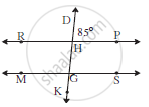In the adjoining figure line RP ||line MS , line DK is a transversal . If ∠DHP = 85° find ∠RHG and ∠HGS.

Chapter:  Co-ordinate Geometry
Concept: General Equation of a Line
1.A.3

∠ACD is an exterior angle of Δ ABC. If ∠B = 40o, ∠A = 70o find ∠ACD.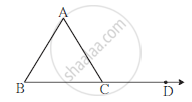Chapter:  Trigonometry
Concept: Trigonometric Ratios of Complementary Angles
1.A.4

Digonals of parallelogram WXYZ intersect at point O. If OY =5, find WY.

Chapter:  Pythagoras Theorem
Concept: Pythagoras Theorem
1.A.5

In which qudrant does point A(-3, 2) lie?
On which axis does point B(12, 0) lie?

Chapter:  Circle
Concept: Tangent Properties - If Two Circles Touch, the Point of Contact Lies on the Straight Line Joining Their Centers
1.A.6

Find the curved surface area of a sphere of radius 1cm. (π = 3.14)

Chapter:  Mensuration
Concept: Surface Area of a Combination of Solids
1.B | Solve any two of the following.
1.B.1

Simplify : 2 sin30 + 3 tan45.

Chapter:  Trigonometry
Concept: Trigonometric Identities
1.B.2

In the adjoining figure, point O is the centre of the cirlcle, seg OM ⊥ chord AB. If OM = 8cm, AB = 12 cm, then find OB.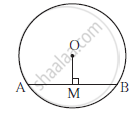Chapter:  Circle
Concept: Angle Subtended by the Arc to the Point on the Circle
1.B.3

In ΔPQR, PQ = 10 cm, QR = 12cm, PR = 8 cm, find the biggest and the smallest angle of the triangle.

Chapter:  Similarity
Concept: Similarity of Triangles
2
2.A | Select the correct alternative answer and write.
2.A.1

How many common tangents can be drawn to two circles which touch each other internally?

(A) One (B) Two (C) Three (D) Four

Chapter:  Circle
Concept: Touching Circles
2.A.2

Distance of point (-3, 4) from the origin is .....
(A) 7 (B) 1 (C) 5 (D) 4

Chapter:  Co-ordinate Geometry
Concept: Distance Formula
2.A.3

sinθ × cosecθ = ?

Chapter:  Trigonometry
Concept: Trigonometric Identities
2.A.4

Measure of an arc of a sector of a circle is 900 and its radius is 7cm. Find the perimeter of the sector.
(A) 44 cm (B) 25 cm (C) 36 cm (D) 56 cm

Chapter:  Mensuration
Concept: Length of an Arc
2.B | Solve any two of the following :
2.B.1

ΔABC ∼ ΔDEF and A(ΔABC) : A Δ(DEF) = 1 : 2 If AB = 4 find DE.

Chapter:  Similarity
Concept: Similarity of Triangles
2.B.2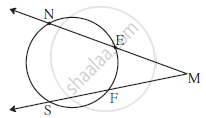In the adjoing figure, m(arc NS) = 1250 m(arc EF) = 37o, find ∠NMS.

Chapter:  Circle
Concept: Cyclic Properties
2.B.3

Find th co-ordinates of the midpoint of the line segment joining P(0, 6) and Q(12, 20).

Chapter:  Co-ordinate Geometry
Concept: Co-ordinates of the Midpoint of a Segment
3
3.A | Carry on any two activities of the following:
3.A.1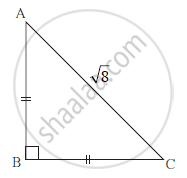With the help of the information given in the figure, fill in the boxes to find AB and BC .

AB = BC                    (Given)

∴∠ BAC = ∠ BCA =
∴ AB = BC =    × AC
× sqrt8
× 2sqrt2
= 2

Chapter:  Similarity
Concept: Similar Triangles
3.A.2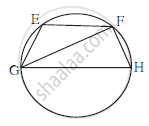In the adjoining figure chord EF || chord GH.
Prove that chord EG ≅ chord FH.
Fill in the boxes and write the complete proof.

Chapter:  Circle
Concept: Inscribed Angle Theorem
3.A.3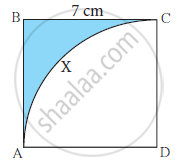Side of square ABCD is 7 cm. With D as the centre and DA as radius, arc AXC is drawn.Find the area of the shaded region with the help of the following flow chart .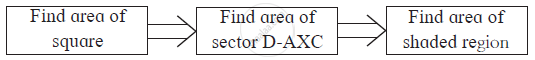Chapter:  Circle
Concept: Theorem - Converse of Tangent at Any Point to the Circle is Perpendicular to the Radius
3.B | Solve any two of the following:
3.B.1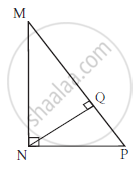In ΔMNP, ∠MNP = 90˚,
seg NQ ⊥ seg MP, MQ = 9,
QP = 4, find NQ.

Chapter:  Pythagoras Theorem
Concept: Pythagoras Theorem
3.B.2

Prove that secθ + tanθ =(costheta)/(1-sintheta).

Chapter:  Trigonometry
Concept: Trigonometric Identities
3.B.3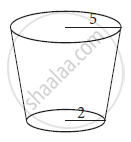Radii of the top and the base of a frustum of a cone are 5 cm and 2 cm respectively. Its height is 9 cm. Find its volume. (π = 3.14)

Chapter:  Trigonometry
Concept: Heights and Distances
4 | Solve any three of the following:
4.A

Prove that :
“If a line parallel to a side of a triangle intersects the remaining sides in two distince points, then the line divides the sides in the same proportion.”

Chapter:  Co-ordinate Geometry
Concept: General Equation of a Line
4.B

Draw a circle with centre O and radius 3.5 cm. Take point P at a distance 5.7 cm from the centre. Draw tangents to the circle from point P.

Chapter:  Geometric Constructions
Concept: Construction of Tangent to the Circle from the Point on the Circle
4.C

Line PQ is parallel to line RS where points P,Q,R and S have
co-ordinates (2, 4), (3, 6), (3, 1) and (5, k) respectively. Find value of k.

Chapter:  Co-ordinate Geometry
Concept: General Equation of a Line
4.D

From the top of a light house, an abserver looking at a boat makes an angle of depression of 600. If the height of the lighthouse is 90 m then find how far is the boat from the lighthouse. (3 = 1.73)

Chapter:  Similarity
Concept: Property of an Angle Bisector of a Triangle
5 | Solve any one of the following.
5.A | Solve any one of the following.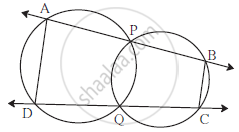Two circles intersect each other at points P and Q. Secants drawn through P and Q intersect the circles at points A,B and D,C. Prove that : ∠ADC + ∠BCD = 180°

Chapter:  Circle
Concept: Tangent Properties - If Two Circles Touch, the Point of Contact Lies on the Straight Line Joining Their Centers
5.B

ΔXYZ ∼ ΔPYR; In ΔXYZ, ∠Y = 60o, XY = 4.5 cm, YZ = 5.1 cm and XYPY = 4/7 Construct ΔXYZ and ΔPYR.

Chapter:  Co-ordinate Geometry
Concept: Concepts of Coordinate Geometry
6 | Solve any one of the following.
6.A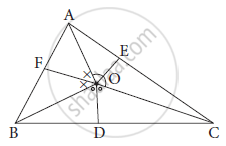O is any point in the interior of ΔABC. Bisectors of ∠AOB, ∠BOC and ∠AOC intersect side AB, side BC, side AC in
F, D and E respectively.
Prove that
BF × AE × CD = AF × CE × BD

Chapter:  Similarity
Concept: Similarity Triangle Theorem
6.B

There is a hemispherical bowl. A cone is to be made such that, if it is filled with water twice and the water is poured in the bowl, it will be filled just completely. State how will you decide the radius and perpendicular height of the cone.

Chapter:  Circle
Concept: Theorem - Converse of Tangent at Any Point to the Circle is Perpendicular to the Radius

## Maharashtra State Board previous year question papers Class 10th Board Exam Geometry with solutions 2018 - 2019

Maharashtra State Board Class 10th Board Exam Geometry question paper solution is key to score more marks in final exams. Students who have used our past year paper solution have significantly improved in speed and boosted their confidence to solve any question in the examination. Our Maharashtra State Board Class 10th Board Exam Geometry question paper 2019 serve as a catalyst to prepare for your Geometry board examination.
Previous year Question paper for Maharashtra State Board Class 10th Board Exam Geometry-2019 is solved by experts. Solved question papers gives you the chance to check yourself after your mock test.
By referring the question paper Solutions for Geometry, you can scale your preparation level and work on your weak areas. It will also help the candidates in developing the time-management skills. Practice makes perfect, and there is no better way to practice than to attempt previous year question paper solutions of Maharashtra State Board Class 10th Board Exam.

How Maharashtra State Board Class 10th Board Exam Question Paper solutions Help Students ?
• Question paper solutions for Geometry will helps students to prepare for exam.
• Question paper with answer will boost students confidence in exam time and also give you an idea About the important questions and topics to be prepared for the board exam.
• For finding solution of question papers no need to refer so multiple sources like textbook or guides.
S